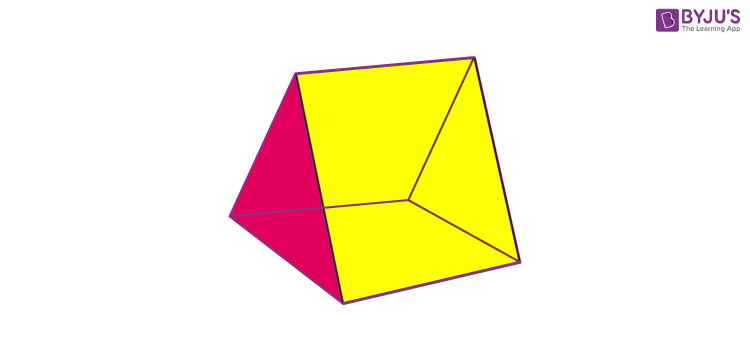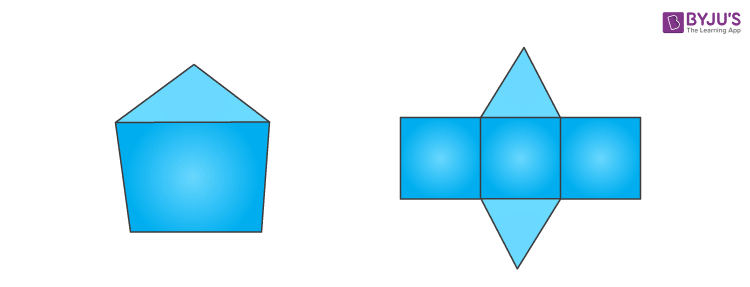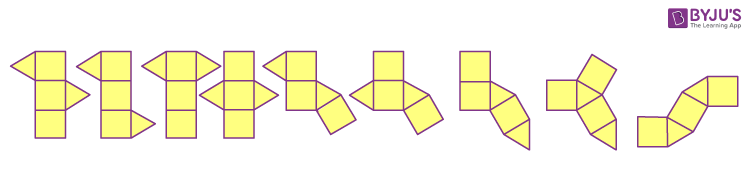Checkout JEE MAINS 2022 Question Paper Analysis : Checkout JEE MAINS 2022 Question Paper Analysis :

# Triangular Prism

A triangular prism is a polyhedron made up of two triangular bases and three rectangular sides. It is a three-dimensional shape that has three side faces and two base faces, connected to each other through the edges. If the sides are rectangular, then it is called the right triangular prism else it is said to be an oblique triangular prism. If the bases are equilateral and sides are squares, then it is called a uniform or regular triangular prism. Like other Prisms, the two bases here are parallel and congruent to each other. In total, it has 5 faces, 6 vertices and 9 edges.

## What is a Triangular Prism?

Triangular Prism is a pentahedron and has nine distinct nets. The edges and vertices of the bases are joined with each other via three rectangular sides. The sides of the triangular prism, which are rectangular in shape are joint with each other side by side. All cross-sections parallel to the base faces are the same as a triangle. A triangular pyramid has four triangular bases unlike the triangular prism, joined with each other and all are congruent to each other.The sides and bases of the triangular prism are congruent or else oblique. The edges of the prism join the corresponding sides.  The two bases of this prism are equilateral triangles and the edges of these triangles are parallel to each other. See the below figure to understand the structure.## Properties of Triangular Prism

Let us discuss some of the properties of the triangular prism.

• It has a total of 9 edges, 5 faces, and 6 vertices (which are joined by the rectangular faces).
• It has two triangular bases and three rectangular sides
• If the triangular bases are equilateral and the other faces are squares, instead of a rectangle, then the triangular prism is said to be semiregular

## Right Triangular Prism

A right triangular prism has its three rectangular sides congruent. Also, the two triangular bases are parallel and congruent to each other. The rectangular or lateral faces are perpendicular to the triangular bases.

## Volume of Triangular Prism

The volume of a triangular prism is equal to the product of the triangular base area and the height of the prism. The formula to find the volume of triangular prism is given by:

 Volume = Area of the Base × Height of prism

Since, the base is in triangular shape, therefore,

Area = ½ b h

Hence,

 Volume of Triangular Prism = ½ × b × h × l

Where b is the base length, h is the height of the triangle and l is the length between the triangular bases.

## Surface Area of Triangular Prism

Surface area of triangular prism is equal to the sum of the lateral surface area and twice the base area of the triangular prism. It is measured in square units. The formula of surface area of triangular prism is given by:

 Surface area of triangular prism = 2A + PH

Where,

• A is the area of the triangular bases,
• P is the perimeter of the bases and
• H is the height of the prism

Now, Area of the triangular base= ½ × b × h

If a, b and c are the sides of the triangular bases, then,

Perimeter of the base = a + b + c

Therefore,

Surface area of triangular prism = 2 (½ × b × h) + (a + b + c)H

 Surface Area of the Triangular Prism = (bh + (a + b + c)H)

Where b and h is the base and height of the bases, respectively and H is the height of the prism.

## Triangular Prism Net

If we open each face of the triangular prism, we will get the net. The net of this prism comprises three rectangles and two triangles. In the below figure you can see the nine distinct nets.## Facts to Remember

 Number of faces = 5 Number of edges = 9 Number of vertices = 6 Shape of the base = Triangular Shape of Sides = Rectangular or Square Surface Area = 2(Area of triangular bases) + Perimeter of base x Height of prism Volume = Area of base x Height of Prism

## Solved Examples on Triangular Prism

Example 1: Find the volume of the triangular prism with base is 5 cm, height is 10 cm, and length is 15 cm.

Solution: Volume of Triangular Prism = ½ × b × h × l

V = ½ × 5 × 10 × 15

Volume, V = 375 cm3

Example 2: If the height of the prism is 4cm and the length of the side of the equilateral triangular base is 6cm. Then find the area of the prism for the above example.

Solutions: We have,

Base = 5cm, height of the base= 10cm, length of the base=15cm, Height of the prism = 4cm

As the base is an equilateral triangle, therefore all its sides will be equal.

Hence, a = b = c = 6cm.

By the formula,

Area of the Triangular Prism = (bh + ( a + b + c)H)

Area = (5 × 10 + (6 + 6 + 6)4)

=(50 + (18)4) = 50 + 72

= 122 cm2

Download BYJU’S App and subscribe with us to get personalised videos and have fun learning.

## Frequently Asked Questions on Triangular Prism

### What is a triangular prism?

A triangular prism is polyhedron and a three-dimensional shape that has 5 faces, 6 edges and 9 vertices. Its bases are triangular and sides are rectangular.

### How many faces, edges and vertices a triangular prism has?

A triangular prism has 5 faces (3 rectangular and 2 triangular), 9 vertices and 6 edges.

### What is the formula to find the volume of a triangular prism?

The formula to find volume of triangular prism is the product of area of triangular base and height of prism.
Volume = ½ × b × h × l

### What is the surface area of a triangular prism?

Surface area of triangular prism is the total area covered by its surface in three dimensional plane. The formula is given by:
Surface area = bh + ( a + b + c)H
Where a, b and c are the sides of triangular bases and H is the height of the prism.

### What is the difference between triangular prism and rectangular prism?

A triangular prism has its bases in triangle shape and a rectangular prism has its bases in rectangle shape.
Quiz on Triangular Prism